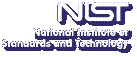Up# Mixing Times of Self-Organizing Lists and Biased Permutations

Amanda P. Streib
Applied and Computational Mathematics Division, NIST

Wednesday, December 12, 2012 15:00-16:00,
Building 101, Lecture Room C
Gaithersburg
Wednesday, December 12, 2012 13:00-14:00,
Room 1-4058
Boulder

Abstract:

Sampling permutations is a fundamental problem from probability theory. One common method is the Nearest-Neighbor Transposition Markov chain ${\cal{M}}_{nn}$, which chooses pairs of adjacent elements $x$ and $y$ uniformly and inverts them with some probability $p_{x,y}$. Fill considered the problem of biased permutations in the context of the Move-Ahead-One list update algorithm and asked for which values of $P= \{p_{x,y}\}$ the chain is rapidly mixing. Only a few special cases are known, including the unbiased case when $p_{x,y} = 1/2$ for all $x,y$, and the constant bias case when $p_{x,y} = p$ for all $x \lt y$ and $p_{x,y} = 1-p$ for $x>y$. Fill conjectured the chain is always rapidly mixing when $P$ is positively biased ( i.e., $p_{x,y} \geq 1/2$ for all $x \lt y$ ) and monotone in both $x$ and $y$, and he verified that for $n\leq 4$, the spectral gap is indeed maximized in the unbiased case.

We use a reduction from biased permutations to Asymmetric Simple Exclusion Processes (ASEPs) to show that the chain may require exponential time to converge in the most general setting, even if $P$ is positively biased. We then prove the chain is rapidly mixing for two general classes, both of which generalize the constant bias case; however, they also include many examples that are not necessarily monotone. Our proofs use bijections between permutations and inversion tables and ASEPs. We then introduce and analyze new Markov chains that are natural in these contexts and use comparison methods to infer polynomial bounds on ${\cal{M}}_{nn}$.

Joint work with: Prateek Bhakta, Sarah Miracle, Dana Randall

Speaker Bio: Amanda is a new NRC postdoc here in the ACMD. She graduated in May 2012 from Georgia Tech with a Ph.D. in Algorithms, Combinatorics, and Optimization with Dana Randall as her advisor. Her research is in randomized algorithms and discrete mathematics. Specifically, she has focused on analyzing the mixing times of Markov chains for sampling and counting, with applications in statistical physics, nanoscience, and computer science.

Presentation Slides: PDF

Contact: B. Cloteaux

Note: Visitors from outside NIST must contact Cathy Graham; (301) 975-3668; at least 24 hours in advance.